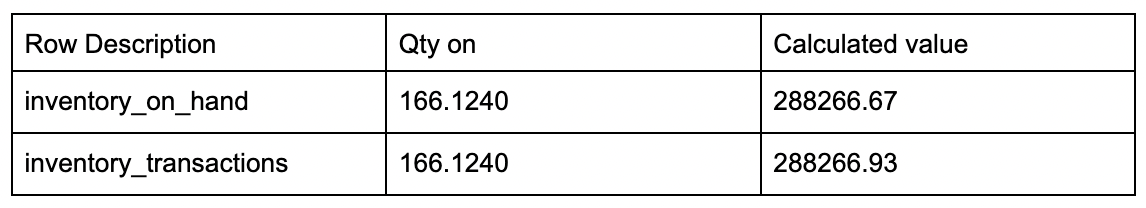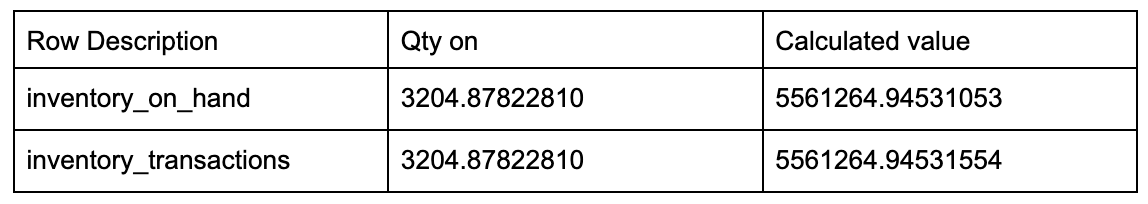##### PostgreSQL and Financial Calculations - Part TwoThe second in a series of blogs covering common mistakes in Database and Application designs for financial calculations.

## Inconsistent precision scaling:

This is probably the most common mistake in database design that I observe. It is understood to use exact data types (such as numeric) and the precision must be fixed, but for whatever reason the decision is made that it’s OK for one table to use numeric(12,4),a second table to use numeric(12,2), and then a third to use numeric(12,6). It’s common to see mixed precision even within the same table. On the surface this does not sound bad, as numeric types are storing the results and math being used is exact. However, this ignores the basic math rule that the least precise number sets the accuracy limit and stack up error.

### Consider 2:

```CREATE TABLE uom_convert(
part_number text,
uom_from text,
uom_to text,
uom_ratio numeric(14,6)
);

CREATE TABLE inventory_on_hand(
part_number text,
uom text,
qty_on_hand numeric(12,4),
cost_per_uom numeric(12,2)
);

CREATE TABLE inventory_transactions(
part_number text,
qty_moved numeric(12,4),
total_moved numeric(12,2)
);

INSERT INTO uom_convert VALUES
('gold', 'grams', 'troyoz', 0.0321507);

INSERT INTO inventory_on_hand VALUES
('gold', 'troyoz', 0, 1735.25 );

truncate inventory_transactions;

INSERT INTO inventory_transactions
(SELECT 'gold', rqty * uom_ratio, --cost_per_uom,
(rqty * uom_ratio) * cost_per_uom
FROM uom_convert
CROSS JOIN (SELECT round((random()*100)::numeric,0)::numeric rqty --using only
integer no fractions values. Fractions values make this look even worse.
FROM generate_series(0,99)) dd
LEFT JOIN inventory_on_hand ON
inventory_on_hand.part_number = uom_convert.part_number
WHERE uom_convert.part_number = 'gold'
AND uom_from = 'grams'
AND uom_to = 'troyoz' );

UPDATE inventory_on_hand SET
qty_on_hand = (SELECT SUM(qty_moved)
FROM inventory_transactions
WHERE part_number = 'gold')
WHERE part_number = 'gold' ;

SELECT 'inventory_on_hand', qty_on_hand, round(qty_on_hand * cost_per_uom,2)
FROM inventory_on_hand where part_number = 'gold'
UNION
SELECT 'inventory_transactions', sum(qty_moved), SUM(total_moved)
FROM inventory_transactions where part_number = 'gold';```Comparing the inventory transaction table value to the actual value, the inventory transactions are over stated by \$0.26 in just 100 transactions. When you run this example you will get different values due to the random() function being used to set the quantity being moved. The returned value will be either higher or lower, however, the inventory_transactions value will always be overstated.

## The solution:

Increasing the precision of the tables. I have come to like numeric(20,8). This gives us a big number: 999 trillion and 8 digits of precision. If the local currency is something akin to Zimbabwe dollars, we will need a larger numeric value. Taking the above example, edit the tables to use numeric(20,8) the result will look something like this.Errors do not start to appear in the calculations until the 6th decimal position. Many consider this level of error contained, as it will take tens of thousands of transactions for this to cause stack up error. In testing, it took a million transactions to create a 0.003 discrepancy between the two tables. This method is sometimes called a Check Digit, where math operations keep an extra digit of precision to avoid truncation or rounding errors.

## Closing Thoughts:

Changing the rounding method not just in PostgreSQL, but throughout the entire application stack wherever calculations are done.